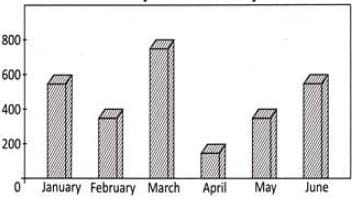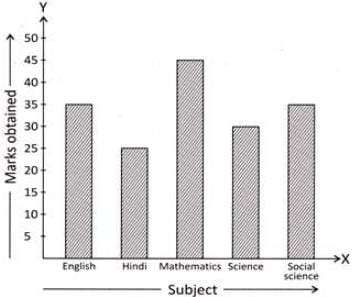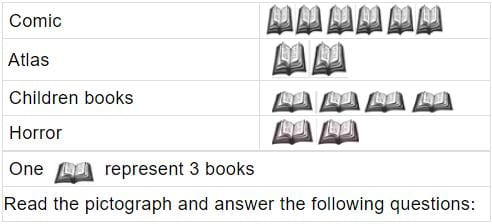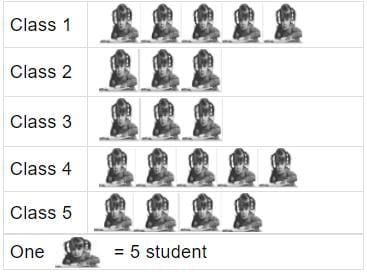# Test: Data handling

## 10 Questions MCQ Test Mathematics for Class 3 | Test: Data handling

Description
Attempt Test: Data handling | 10 questions in 15 minutes | Mock test for Class 3 preparation | Free important questions MCQ to study Mathematics for Class 3 for Class 3 Exam | Download free PDF with solutions
QUESTION: 1

### Production of bicycles at a bicycle manufacturing unit in six months is given below. In which month the product of the company was maximum?Solution:

Option (b) is correct. Seeing the graph, we find that the height of bar shown for the month of March is the highest and it is at 800. Therefore, the production of company was maximum in March. Rest of the options is incorrect because of the correctness of option (b).

QUESTION: 2

### Direction: Following bar graph tells us about the marks obtained by Manju in five different subjects in a particular test.Read the Bar graph and answer the following question: In which subject manju scored more than 40 marks?

Solution:

Option (b) is correct. Seeing the bar graph, we find that in Mathematics, Manju scored more than 40 marks that is 45 marks. Rest of the options is incorrect because of the correctness of option (b).

QUESTION: 3

### Direction: Following bar graph tells us about the marks obtained by Manju in five different subjects in a particular test.Read the Bar graph and answer the following question: If 20 was the passing marks, in which subject did Manju fail?

Solution:

Option (e) is correct. Passing marks were 20 and Manju scored the least marks in Hindi that is 25 marks. Therefore, Manju did not fail in any subject. Rest of the options is incorrect because of the correctness of option (e).

QUESTION: 4

Direction: Following bar graph tells us about the marks obtained by Manju in five different subjects in a particular test.In which two subjects did Manju score equal marks?

Solution:

Option (d) is correct. Seeing the bar graph, we find that Manju scored equal marks in English and Social Science that is 35 marks. Rest of the options is incorrect because of the correctness of option (d).

QUESTION: 5

Direction: Following bar graph tells us about the marks obtained by Manju in five different subjects in a particular test.If 50 was the maximum marks in each subject, what marks did Manju score in English, Mathematics and Science out of 150?

Solution:

Option (b) is correct. Sum of marks scored in English, Mathematics and Science =35+45+30=110 Therefore, Manju scored 110 marks out of 150 in English, Mathematics and Science. Rest of the options is incorrect because of the correctness of option (b).

QUESTION: 6

Direction: Following pictograph tells us about the kind of books read by Prashant.How many children books did Prashant read?

Solution:

One book is represent 3 books
So 4 book is represent is = 4 x 3 = 12
So 12 many children books did Prashant read.

QUESTION: 7

Direction: Following pictograph tells us about the kind of books read by Prashant.Which two types of books that Prashant read were same in number?

Solution:

In this Figure Atlas book is 2 books
And same is Horror book is 2 books
So Altas and Horror of books that Prashant read were same in number.

QUESTION: 8

Direction: Following pictograph tells us about the kind of books read by Prashant.How many more books on comics than Horror books are read by Prashant?

Solution:
QUESTION: 9

Direction: Following pictograph tells us about the kind of books read by Prashant.How many books did Prashant read in all?

Solution:

One book is represent 3 books
Soi in this figure total books is 14
= 14 x 3 = 42
So correct ans is option A.

QUESTION: 10

Given pictograph represents the number of students in class 1 to 5 of a school.How many students are there in all?

Solution:

One student is represent 5 students
So in this figure total students is 20
= 20 x 5 = 100
So correct ans is option C.Use Code STAYHOME200 and get INR 200 additional OFF Use Coupon Code